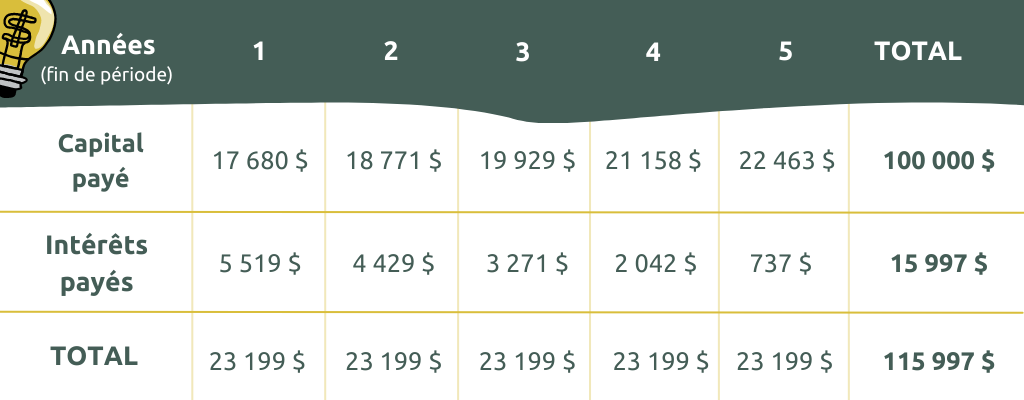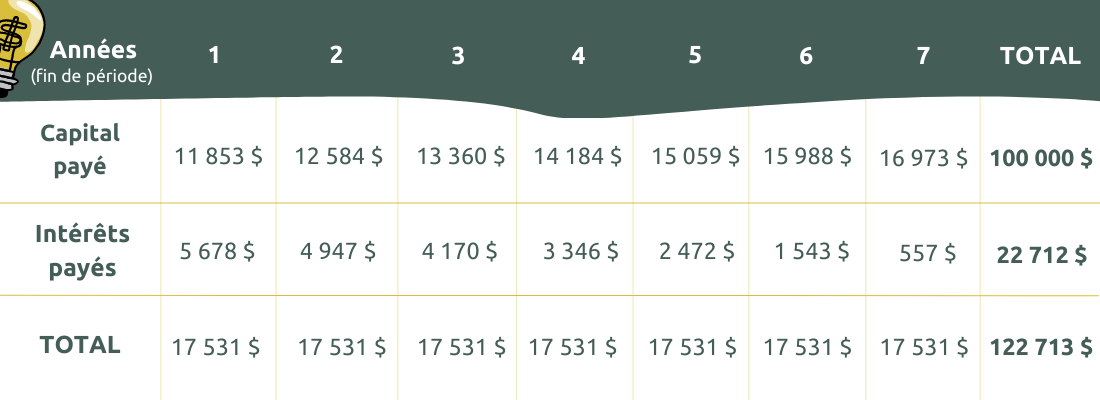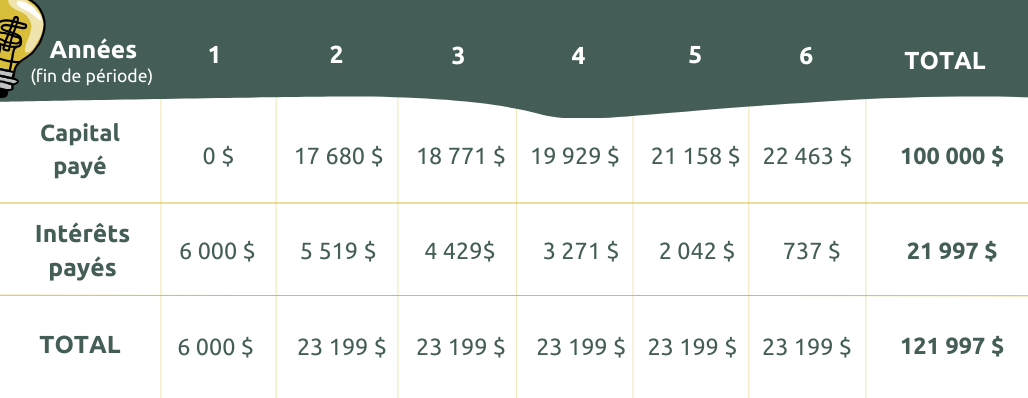# The capital importance of distinguishing between interest and capital

### What is an interest rate, from a borrower’s perspective?

The interest rate is the cost of borrowing money. There are generally three types:

• Variable rate: A rate that can change over the duration of the loan, because it depends on the economic context at any given time; it can go up or down.
• Fixed rate: A rate that does not change over the duration of the loan, and is fixed at the time of signing the loan agreement.
• Mixed rate: A combination of variable and fixed rate that can be fixed for several years and then become variable afterwards.

### What is an interest rate made up of?

The interest rate reflects the risk a lender takes when lending money.
The riskier the loan, the higher the rate. Conversely, the less risky the loan,
the lower the rate.

This is why, most of the time, a start-up will pay a much higher rate than a company that has been established for several years. In general, rates are calculated based on the prime rate plus a risk premium.

The prime rate is the rate at which a commercial bank lends to its least risky customers. For its part, the risk premium corresponds to the specific risk of a project or company.

### How much does a loan cost?

In this section, we will look at the amount of interest paid on a loan, the impact of extending the amortization period, and the impact of a moratorium on capital.

In the vast majority of cases, the fixed payment made on a loan is made up of an “interest” portion and a “capital” portion.

The interest is calculated on the balance that remains at the beginning of each month. As the balance of the loan decreases each month, the interest paid also decreases, while the capital portion increases.

#### Example 1

\$100,000 loan over 5 years at a 6% interest rate with monthly repayments:We can see that the interest payment decreases over time and the capital payment increases.

#### Example 2

\$100,000 loan over 7 years at a 6% interest rate with monthly repayments:In this case, extending the loan by 2 years will increase the total interest paid; on the other hand, the monthly payments will decrease.

#### Example 3

\$100,000 loan over 6 years at a 6% interest rate with monthly repayments and a one-year moratorium on capital at the beginning of the loan:Note here that there is no repayment of capital in the first year, which results in paying interest on the same balance for the first twelve months. During this first year, the balance of the loan does not decrease, and this results in a significant increase in the total interest paid.

### Takeaways

In conclusion, the choice of the terms of a loan can strongly influence the cost of the loan, but also liquidity.

Extending the term of a loan or including a moratorium on principal will result in a higher or lower cost. On the other hand, payments will decrease, so the company will have less pressure on its liquidity.

If the company has the liquidity and pays back the loan quickly, the loan will be less expensive.